# Gases, Liquids, and Solids Flashcards Preview

## Chemistry 101 > Gases, Liquids, and Solids > Flashcards

Flashcards in Gases, Liquids, and Solids Deck (48):
1

2

3

4

5

6

## What phase is the substance in sections A, B, and C in the below diagram?### A represents the Solid phase region. B represents the Liquid phase region. C represents the Gas phase region.7

## What phase conversions are being shown with arrows A and B?### A is Fusion (or Melting) B is Freezing (or Solidification)8

## What phase conversions are being shown with arrows A and B?### A is Vaporization (or boiling) B is Condensation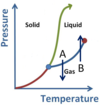9

## What phase conversions are being shown with arrows A and B?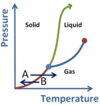### A is Sublimation B is Deposition10

## What points are arrows A and B pointing to?### A is the Triple Point. B is the Critical Point. Note: though the concept of Plasma exists, the AP Chem exam does not explicitly test this as a phase.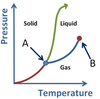11

12

13

## How does the phase diagram of water differ from the one below?### Water has a slightly negative sloped Solid/Liquid boundary.  This is due to water's Solid phase being less dense than its Liquid phase.14

15

16

17

18

19

20

21

22

23

24

## What processes are associated with the plateaus A and B, for the general heating curve below?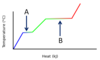### At A, fusion is occurring. Hf is used to calculate the heat needed for plateau A. At B, vaporization is occurring. Hv is used to calculate the heat needed for plateau B.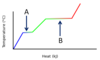25

## Why are the slopes zero for plateaus A and B?### Zero slope means no temperature change. This occurs at these times because any heat input is dedicated to breaking bonds between molecules, instead of increasing temperature. Ex: during the vaporization of water, heat is needed to break the hydrogen bonds between liquid water molecules and convert it to gaseous steam.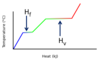26

## What equation determines the heat added in the sections where the temperature is rising in the below diagram?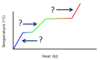### q = mcΔT This formula calculates heat required to raise a certain mass of a substance by a change in temperature ΔT. where: q = heat required in J m = the sample's mass in g c = the substance's specific heat in J/g*K ΔT = temperature change in K27

28

## What is the equation to calculate heat required when at the plateaus in the phase change diagram below?### q = mHL This formula calculates heat required to change a certain mass of a substance from one phase to another. HL would be Hf for the first plateau and Hv for the second plateau. where: q = heat required in J m = mass in g HL = latent heat of phase change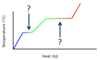29

30

31

32

## Charles's Law

### Charles' Law states that the volume of a gas is directly proportional to temperature while at a constant pressure. Equation:33

34

## Boyle's Law

### Boyle's Law states that the volume of a gas is inversely proportional to its pressure while at a constant temperature. Equation: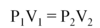35

36

37

38

39

40

41

42

43

44

45

46

47

48